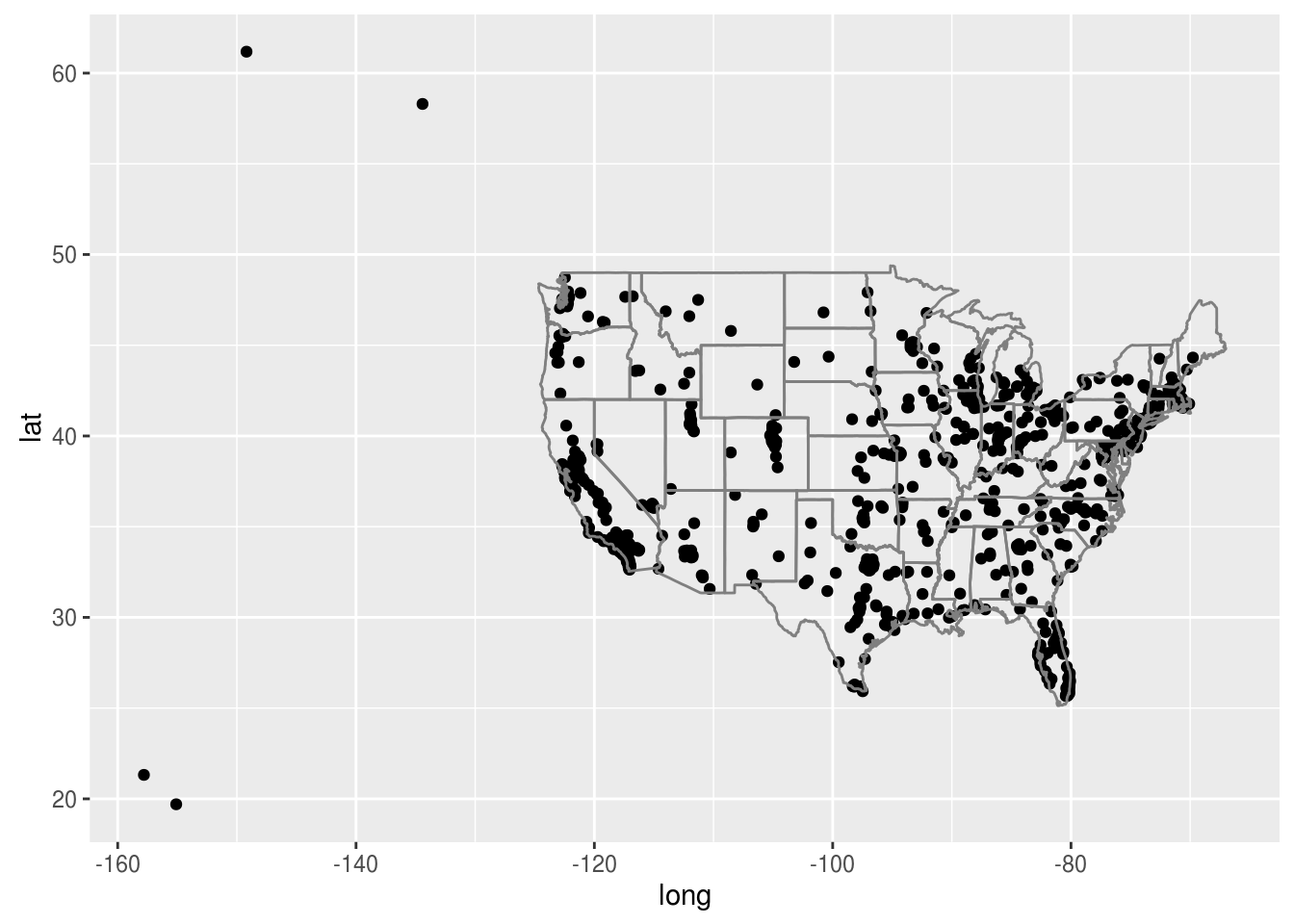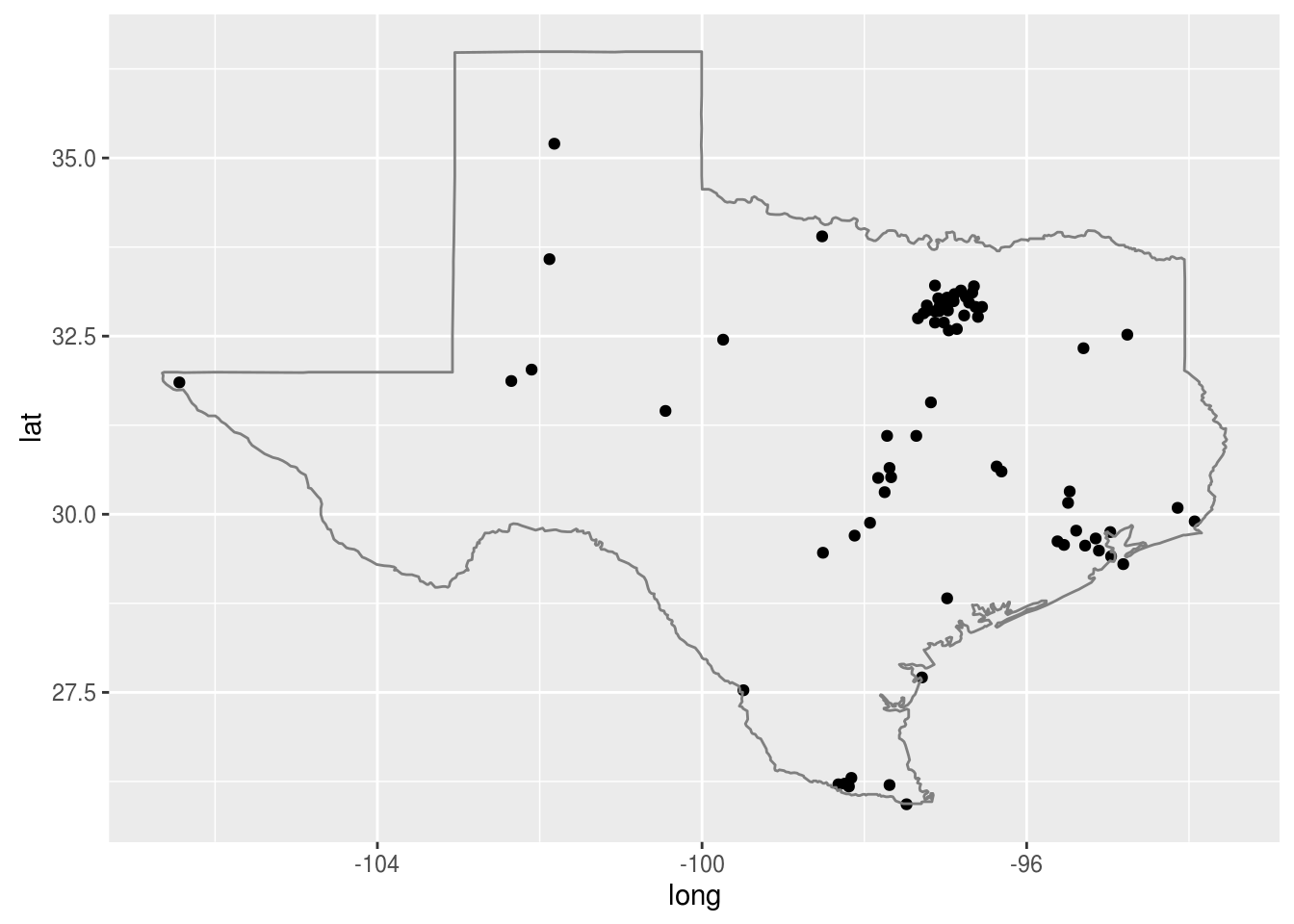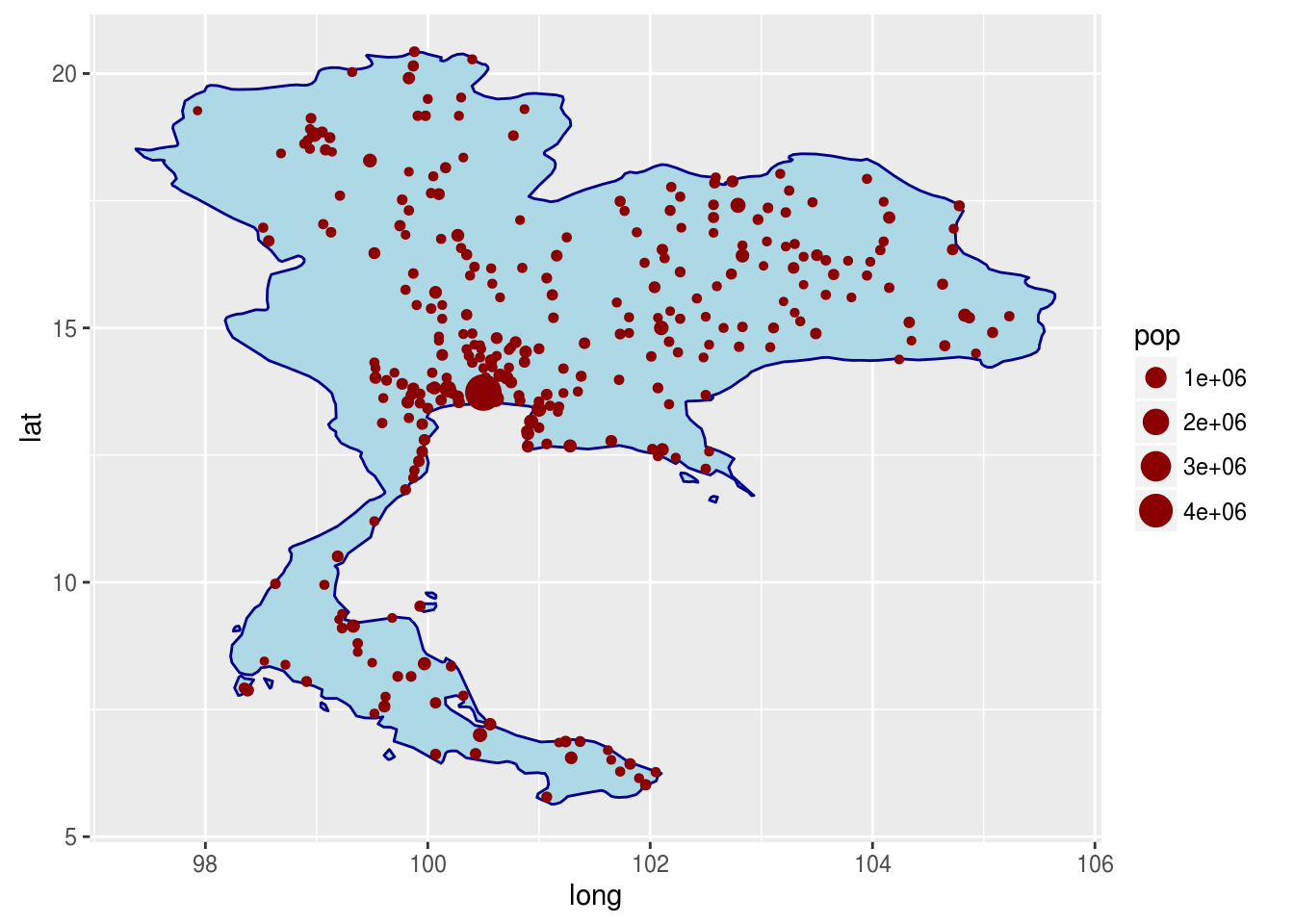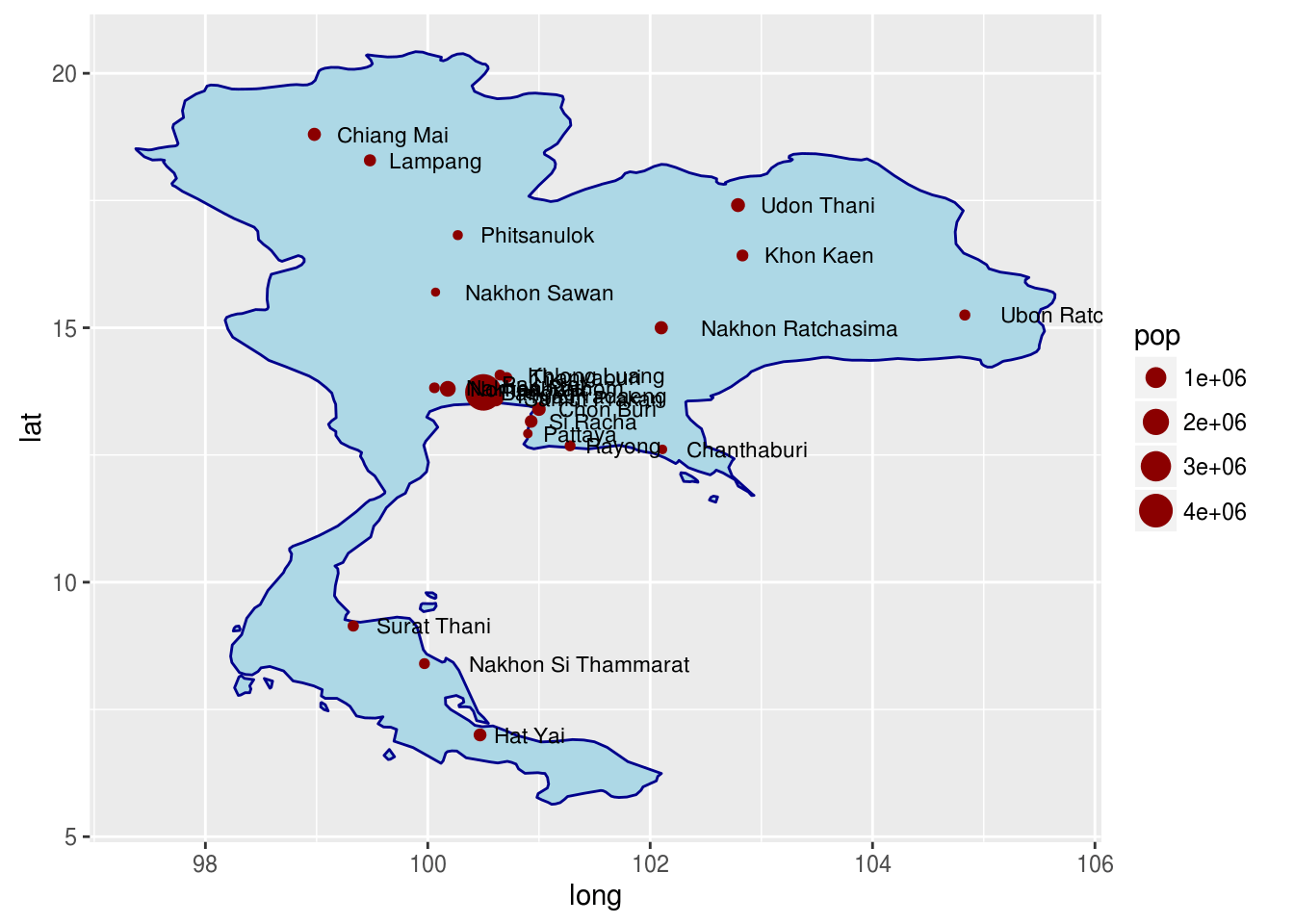# Using Maps in ggplot2

It seems as though there are no limits to what can be done with ggplot2. Another example of this is the use of maps in presenting data. If you are trying to share information that depends on location then this is an important feature to understand.

This post will provide some basic explanation for understanding how to use maps with ggplot2.

The Maps Package

One of several packages available for using maps with ggplot2 is the “maps” package. This package contains a limited number of maps along with several databases that contain information that can be used to create data-filled maps.

The “maps” package cooperates with ggplot2 through the use of the “borders” function and plotting the plot using lattitude and longitude for the “aes” function. After you have installed the “maps” package you can run the example code below.

``````library(ggplot2);library(maps)
ggplot(us.cities,aes(long,lat))+geom_point()+borders("state")``````In the code above we told R to use the data from “us.cities” which comes with the “maps” package. We then told R to graph the latitude and longitude and to do this by placing a point for each city. Lastly, the “borders” function was use to place this information on the state map of the US.

There are several points way off of the map. These represents datapoints for cities in Alaska and Hawaii.

Below is an example that is limited to one state in America. To do this we first must subset the data to only include one state.

``````tx_cities<-subset(us.cities,country.etc=="TX")
ggplot(tx_cities,aes(long,lat))+geom_point()+borders(database = "state",regions = "texas")``````The map shows all the cities in the state of Texas that are pulled form the “us.cities” dataset.

We can also play with the colors of the maps just like any other ggplot2 output. Below is an example.

``````data("world.cities")
Thai_cities<-subset(world.cities, country.etc=="Thailand")
ggplot(Thai_cities,aes(long,lat))+borders("world","Thailand", fill="light blue",col="dark blue")+geom_point(aes(size=pop),col="dark red")``````In the example above, we took all of the cities in Thailand and saved them into the variable “Thai_cities”. We then made a plot of Thailand but we played with the color and fill features. Lastly, we plotted the population be location and we indicated that the size of the data point should depend on the size. In this example, all the data points were the same size which means that all the cities in Thailand in the dataset are about the same size.

We can also add text to maps. In the example below, we will use a subset of the data from Thailand and add the names of cities to the map.

``````Big_Thai_cities<-subset(Thai_cities, pop>100000)
ggplot(Big_Thai_cities,aes(long,lat))+borders("world","Thailand", fill="light blue",col="dark blue")+geom_point(aes(size=pop),col="dark red")+geom_text(aes(long,lat,label=name),hjust=-.2,size=3)``````In this plot there is a messy part in the middle where Bangkok is a long with several other large cities. However, you can see the flexiability in the plot by adding the “geom_text” function which has been discussed previously. In the “geom_text” function we added some aesthetics as well add the “name” of the city.

Conclusion

In this post, we look at some of the basic was of using maps with ggplot2. There are many more ways and features that can be explored in future post.